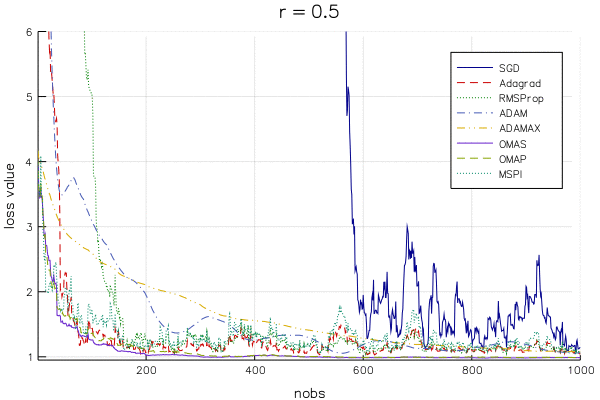# Choosing a Learning Rate is Hard

Stochastic approximation (SA) algorithms have a wide variety of uses in machine learning and big data. In the SA setup, there's function you're trying to minimize,

$F(\theta) = E_Y[f(Y,\theta)],$

but you're unable (or it's expensive) to evaluate this expectation. Instead of working with $F$ directly, we'll use random samples from the distribution of $Y$ ($y_1,y_2,\ldots$) and take small steps for our estimate $\theta^{(t)}$ that we think will improve (decrease) the value of $F(\theta)$.

As a concrete example, think of linear regression:

$F(\beta) = \sum_{i=1}^n \frac{1}{2}(y_i - x_i^T\beta)^2.$

We need to make updates to our estimate $\beta^{(t)}$ given a single observation $(y_t, x_t)$. The SA approach is extendable to mini-batches of multiple observations, but we'll stay in the single-observation case for now.

On-line updates for linear regression have a closed form (which I will blog about sometime in the future), but pretend we don't know this and we're going to apply stochastic gradient descent (SGD), parameterized by a learning rate $\gamma_t$.

• SGD (General form)

$\theta^{(t)} = \theta^{(t-1)} - \gamma_t \nabla f(y_t, \theta^{(t-1)}).$
• SGD for Linear Regression:

$\beta^{(t)} = \beta^{(t-1)} + \gamma_t (y_t - x_t^T\beta^{(t-1)})x_t.$

This learning rate is often chosen to be $t^{-r}$ where $r \in [.5, 1]$.

## What Learning Rate is "Best"?

Below is an animation of the linear regression loss value ($F(\beta)$) for a changing learning rate parameter (from $.5$ to $1$) for SGD and variety of variants that supposedly improve on SGD (hyperparameters chosen as recommended by the papers that introduced the algorithm). The y-axis is cut off at the loss evaluated at the OLS estimate, so that as the lines approach the bottom of the plot, the algorithm approaches the best solution.Note that you won't find literature on OMAS, OMAP, and MSPI, as they are introduced in my dissertation (a paper or two is still in the works).

It is difficult to pick out a clear winner (except for maybe MSPI...) for a few reasons:

1. The methods are extremely dependent on the learning rate

2. The methods react to the learning rate in different ways

The takeaway is that we can't make definitive statements about which method is best for even a straightforward model like linear regression, so be wary of bold claims about a given method's superiority in deep learning. It seems to me that one should be very concerned with an algorithm's "robustness" to the choice of learning rate, which is something that doesn't get much attention in the SA literature.